# Popsicles

Francis went to buy ice lollies. If he buy 8 popsicles he missed 4 USD. When he buy 7 popsicles, got back 1 USD.

How many USD was a popsicle?

Correct result:

p =  5

#### Solution: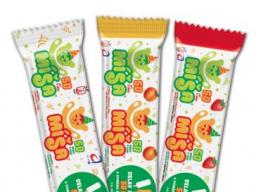We would be pleased if you find an error in the word problem, spelling mistakes, or inaccuracies and send it to us. Thank you!Tips to related online calculators
Do you have a linear equation or system of equations and looking for its solution? Or do you have quadratic equation?

## Next similar math problems: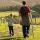Daddy is a 46 year old and son is at age of 16. When (which year) was/will daddy 5 times more years than his son?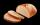Several pieces of bread were in the store. Half of them plus a half of bread bought a cookbook. Of the remaining bread, half of it plus half of the bread was bought by Mr. Novák. The last bread was bought by Ms. Small. How many breads were in the store at
• Bricks II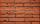3/4 bricks weighs 6 kg and 2/3 bricks. How weighs one whole brick?
• Chewing gums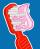For 3 chewing gums, you will pay 20 CZK less than 7 chewing gums. How much is 1 chewing gum and how much does a 5pcs package cost?
• Apples 3Julka has 5 apples more than Hugo and four apples less than Annie. Hugo has 17 apples. How many apples has Julka and how Annie?
• Class alphabet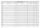All 29 pupils in the class are written in a class by alphabet. The number of pupils enrolled before Paul is three times higher than the number of pupils behind him. Calculate how many pupils are enrolled after Paul.
• Trains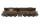Daily passes the same track section 8 pairs of trains with average 2400 passagers. How many people average travel in one train?
• Tickets 3A total of 645 tickets were sold for the school play. They were either adult tickets or student tickets. There were 55 fewer student tickets sold than adult tickets. How many adult tickets were sold?
• Equation with one variableSolve the following equation with one unknown: 5(7s + 5) =130
• Forest nurseryIn the forest nursery after winter, they found that 1/10 stems died out of them. For them, they land 193 new spruces. How many spruces are in the forest nursery?
• CagesHonza had three cages (black, silver, gold) and three animals (guinea pig, rat and puppy). There was one animal in each cage. The golden cage stood to the left of the black cage. The silver cage stood on the right of the guinea pig cage. The rat was in th
• Eq1Solve equation: 4(a-3)=3(2a-5)
• Simple equation 1035= 7*3*x what is x?
• If-then equationIf 5x - 17 = -x + 7, then x =
• Six te 2If 3t-7=5t, then 6t=
• Simple equation 8Solve the following equation: 36=-(1+7x)-6(-7-x)Added together and write as decimal number: LXVII + MLXIV